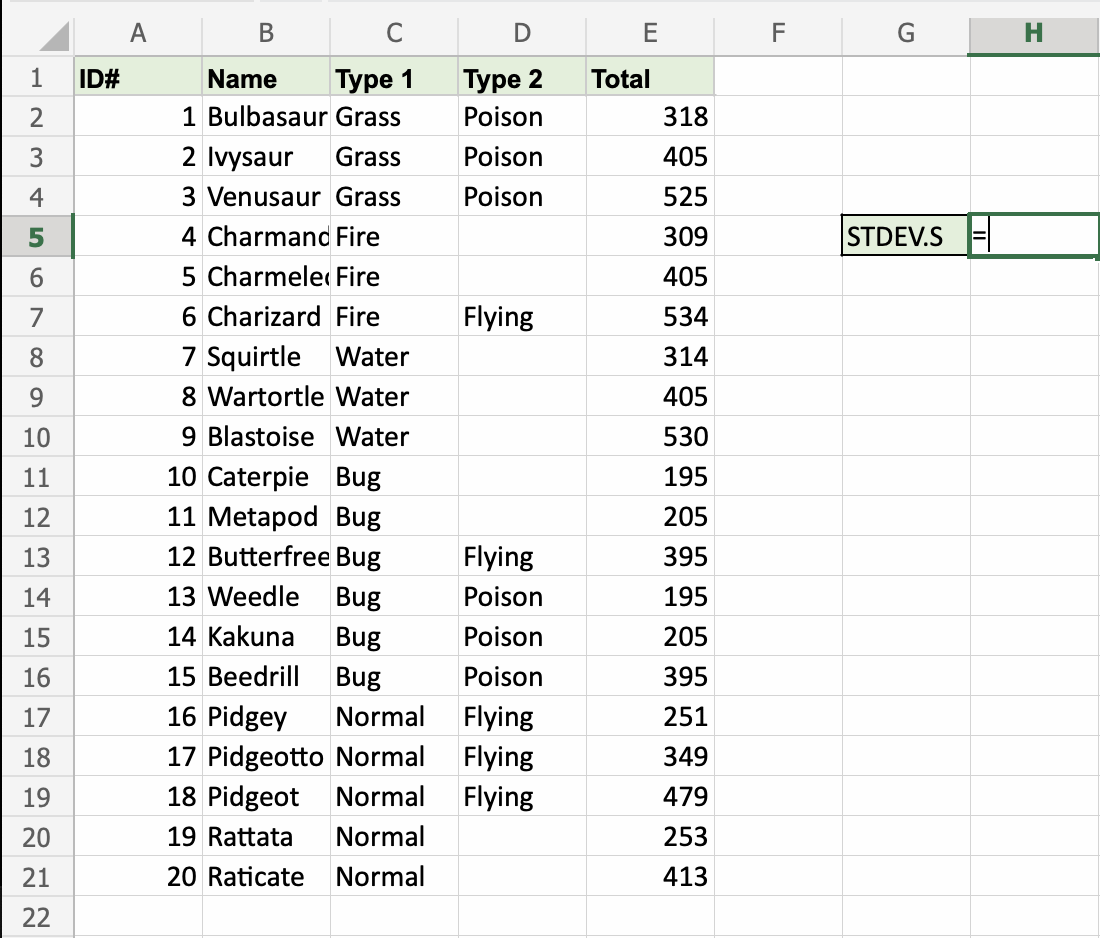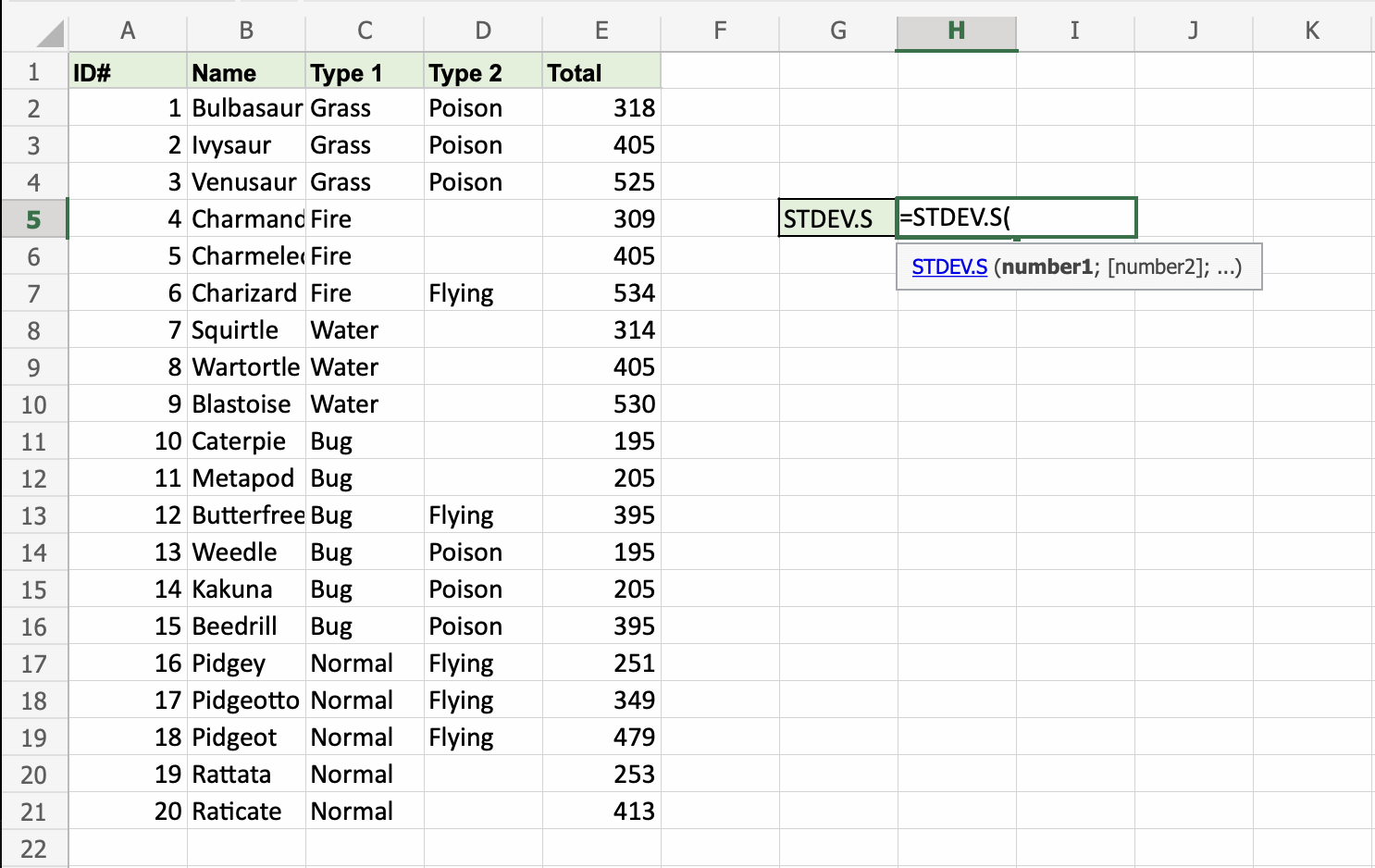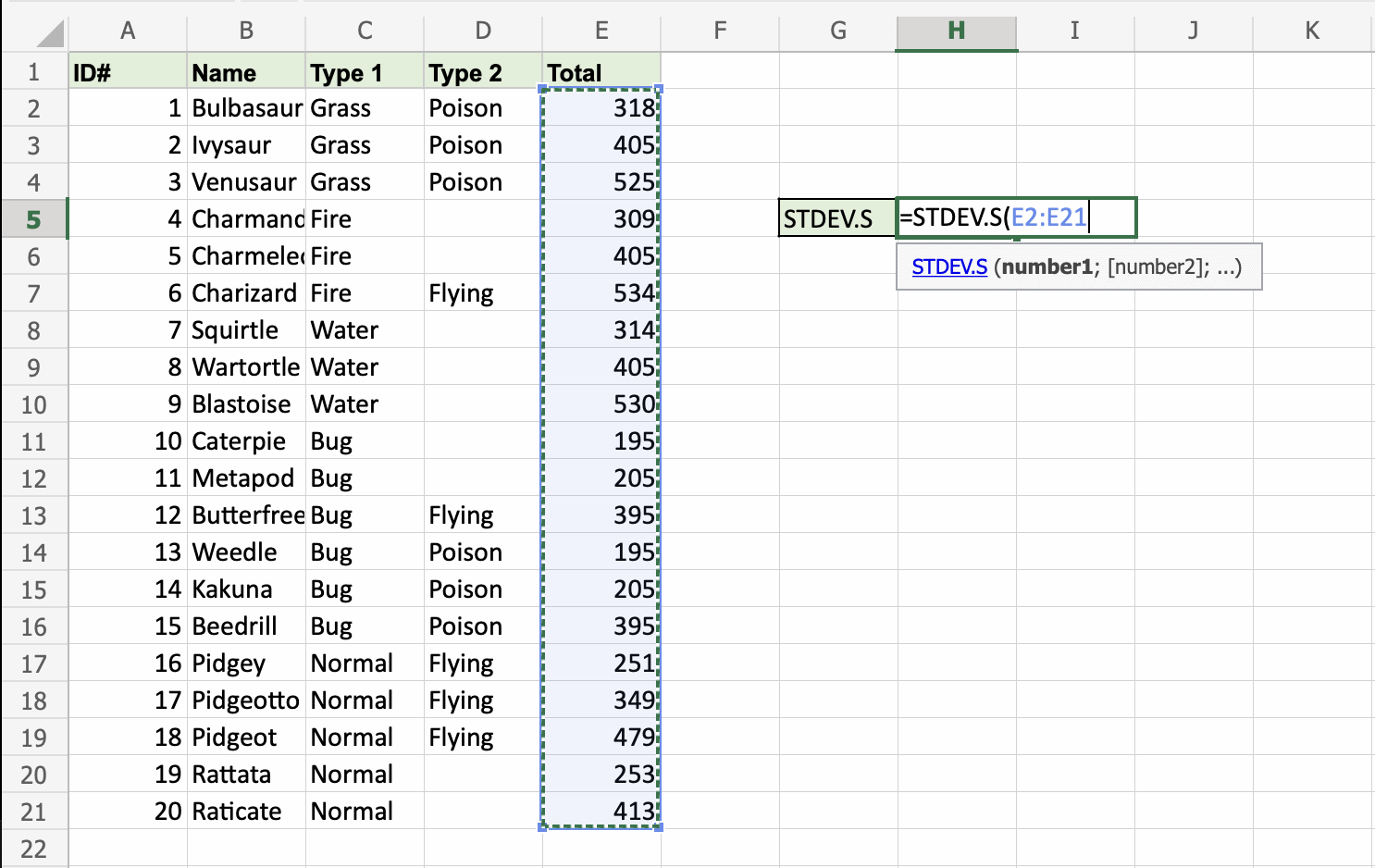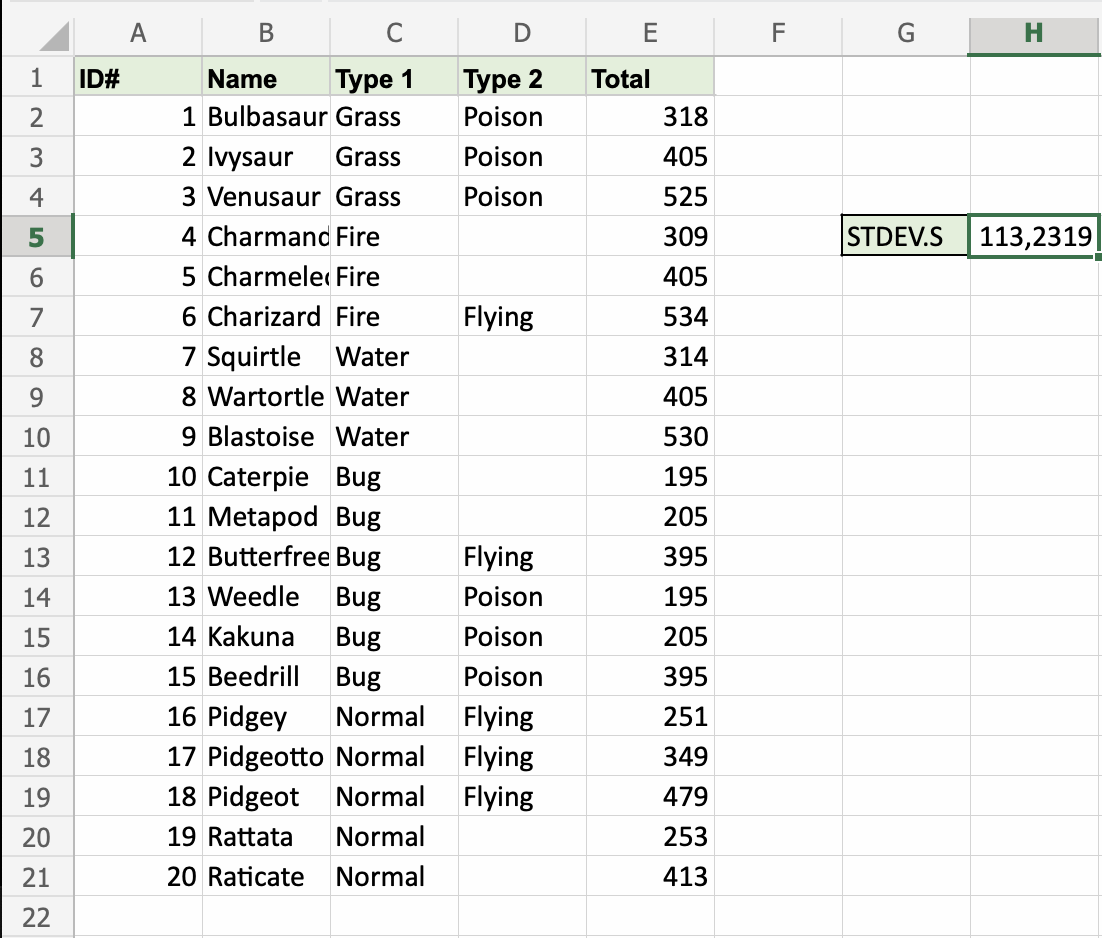# Excel STDEV.S Function

## STDEV.S Function

The STDEV.S function is a premade function in Excel, which calculates the Standard Deviation (Std) for a sample.

It is typed `=STDEV.S`

Note: This function ignores cells with text and logic.

Note: Standard deviation (σ) measures how far a 'typical' observation is from the average of the data (μ). You can read more about standard deviation in our Statistics - Standard Deviation Tutorial.

Tip: There is another function called STDEV.P that can be used if you have the data for the entire population.

How to use the `=STDEV.S` function:

1. Select a cell (`H5`)
2. Type `=STDEV.S`
3. Double click the STDEV.S command
4. Select a range (`E2:E21`)
5. Hit enter

Let's have a look at an example!

Find the Standard Deviation for the sample of Pokemon total stats in the range `E2:E21`:Copy ValuesThat's the way! The STDEV.S function has successfully returned the Standard Deviation `113,2319` for the sample of Pokemons Total stats.